## What are basic JavaScript mathematical operators?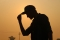Published on 16-Feb-2018 07:42:51
The basic mathematical operators in JavaScript include the following −Arithmetic OperatorsComparison OperatorsLogical (or Relational) OperatorsAssignment OperatorsLet’s see how Arithmetic operators work and what does it include −Sr.NoOperator and Description1+ (Addition)Adds two operandsEx: A + B will give 302- (Subtraction)Subtracts the second operand from the firstEx: A - B will give ... Read More

## What are C++ Floating-Point Constants?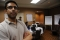Published on 16-Feb-2018 07:26:49
Floating-point constants specify values that must have a fractional part.Floating-point constants have a "mantissa, " which specifies the value of the number, an "exponent, " which specifies the magnitude of the number, and an optional suffix that specifies the constant's type(double or float).The mantissa is specified as a sequence of ... Read More

## What are C++ Integer Constants?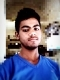Published on 16-Feb-2018 07:21:07
Integer constants are constant data elements that have no fractional parts or exponents. They always begin with a digit. You can specify integer constants in decimal, octal, or hexadecimal form. They can specify signed or unsigned types and long or short types.In C++ you can use the following code to ... Read More

## What are JavaScript Math Functions?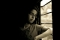Published on 16-Feb-2018 07:30:28
The following are some of the Math Functions in JavaScript −S.NoMethod & Description 1abs()Returns the absolute value of a number.2acos()Returns the arccosine (in radians) of a number.3asin()Returns the arcsine (in radians) of a number.4atan()Returns the arctangent (in radians) of a number.5atan2()Returns the arctangent of the quotient of its arguments.6ceil()Returns the smallest ... Read More

## How to get the tangent of a number in JavaScript?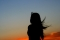Published on 16-Feb-2018 07:25:04
To get the tangent of a number in JavaScript, use the Math.tan() method. This method returns the tangent of a number. The tan method returns a numeric value that represents the tangent of the angle.ExampleYou can try to run the following code to get the tangent of a number −<html> ... Read More

## What is the difference between literal and constant in C++?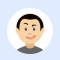Published on 16-Feb-2018 07:50:34
A literal is a value that is expressed as itself. For example, the number 25 or the string "Hello World" are both literals.A constant is a data type that substitutes a literal. Constants are used when a specific, unchanging value is used various times during the program. For example, if ... Read More

## How to get the square root of a number in JavaScript?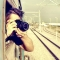Published on 16-Feb-2018 07:17:49
To get the square root, use the Math.sqrt() method. This method returns the square root of a number. If the value of a number is negative, sqrt returns NaN.ExampleYou can try to run the following code to get the square root of a number −<html>    <head>       ... Read More

## How to get the sine of a number in JavaScript?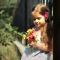Published on 16-Feb-2018 07:18:15
To get the sine of a number, use the JavaScript sine() technique. The sin technique returns a numeric value between -1 and one, that represents the sine of the argumentExampleYou can try to run the following code to get the sine of a number in JavaScript −<html>    <head>   ... Read More

## What are literals in C++?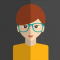Published on 16-Feb-2018 07:15:32
A literal is any notation for representing a value within the source code. They just exist in your source code and do not have any reference a value in memory. Contrast this with identifiers, which refer to a value in memory.There are several types of literals in C++. Some of ... Read More

## How to get a pseudo-random number between 0 and 1 in JavaScript?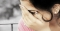Published on 16-Feb-2018 07:14:35
To get a pseudo-random number between 0 and 1, use the Math.random() method in JavaScript.ExampleYou can try to run the following code to get a pseudo-random number between 0 and 1 −<html>    <head>       <title>JavaScript Math random() Method</title>    </head>    <body>       <script>   ... Read More